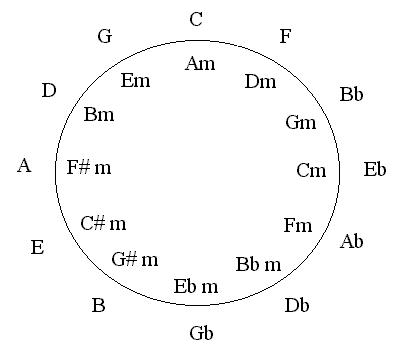# GuitarScholar.co.uk

## Circle of Fifths

The diagram below is called the circle of fifths (otherwise known as the circle of fourths). The numbers around the outside of the circle are ordered so that every step in a clockwise direction takes you up a fourth and every step in an anticlockwise direction takes you up a fifth.

For example, if E is the root note and we take one step anticlockwise then we land on B, which is a fifth higher (these two notes would make up a power chord). If instead we took a step in a clockwise direction we would land on A, which is a fourth higher.Using the circle of fifths to spot chord families

 I II III IV V VI VII C D E F G A B

If we are working with the C major scale we can look at C (I) on the circle of fifths diagram and see that the nearest two positions are F (IV) and G (V), which make up the immediate family of chords. The next two nearest chords are Bb (bVII) and D (II), (the next most commonly associated chords).

Around the inside of the circle lay the relative minors. Each relative minor chord is a minor third below the relative major (A is three frets below C). The relative minor chords can be used to extend the immediate family of chords surrounding C.

Using the circle of fifths to transpose chord progressions

To transpose means to move chords or chord progressions into a different key. If we're in the key of C and are using the chords C (I), F (IV), G (V) and Am (vi), we can change to the key of G simply by moving all chords one step anticlockwise. This would leave us with the chords: G (I), C (IV), D (V) and Em (vi).